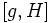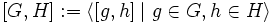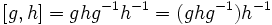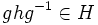Equivalence of conjugacy and commutator definitions of normality

This article gives a proof/explanation of the equivalence of multiple definitions for the term normal subgroup
View a complete list of pages giving proofs of equivalence of definitions

Statement

Suppose$H$ is a subgroup of a group$G$ and$g \in G$ is an element. Then the following are equivalent:

1.$gHg^{-1} \subseteq H$.
2. The commutator$[g,H] \subseteq H$, where$[g,H]$ is the subgroup generated by$[g,h] = ghg^{-1}h^{-1}, h \in H$.

If these equivalent conditions hold for all$g \in G$, then$H$ is a normal subgroup of$G$. Note that the second condition on all$g \in G$ would translate to saying that$[G,H] := \langle [g,h] \mid g \in G, h \in H\rangle$ is contained in$H$.

Note that$gHg^{-1} \subseteq H$ is a weaker condition than$gHg^{-1} = H$ for a particular$g \in G$.

More on the proof techniques

The survey article manipulating equations in groups discusses similar proof techniques involving equations that deal with elements and subsets of groups.

Proof

(1) implies (2)

Given: Group$G$, subgroup$H$,$g \in G$ such that$gHg^{-1} \subseteq H$.

To prove:$[g,H] \subseteq H$.

Proof: Since$H$ is a subgroup, it suffices to show that all the generators of$[g,H]$ are in$H$, i.e., that$[g,h] \in H$ for every$h \in H$. For this, note that:$\! [g,h] = ghg^{-1}h^{-1} = (ghg^{-1})h^{-1}$

By assumption, since$h \in H$$ghg^{-1} \in H$, and so the quotient$(ghg^{-1})h^{-1}$ is also in$H$. This completes the proof.

(2) implies (1)

Given: Group$G$, subgroup$H$,$g \in G$ such that$[g,H] \subseteq H$.

To prove:$gHg^{-1} \subseteq H$.

Proof: For any$h \in H$, we have:$\! ghg^{-1} = (ghg^{-1}h^{-1}h = [g,h]h$

By assumption, both$[g,h]$ and$h$ are in$H$, hence$ghg^{-1} \in H$.